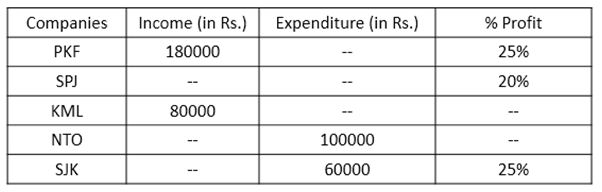# IBPS RRB Clerk Prelims Quantitative Aptitude Questions 2019 (Day-15)

Dear Aspirants, Our IBPS Guide team is providing new series of Quantitative Aptitude Questions for IBPS RRB Clerk Prelims 2019 so the aspirants can practice it on a daily basis. These questions are framed by our skilled experts after understanding your needs thoroughly. Aspirants can practice these new series questions daily to familiarize with the exact exam pattern and make your preparation effective.

### Click Here to Subscribe Crack High Level Puzzles & Seating Arrangement Questions PDF 2019 Plan

[WpProQuiz 6816]

Directions (1 – 5): In each of these questions, one term in the given number series is wrong. Find out the wrong term.

1) 5, 87, 600, 640, 867, 893

a) 867

b) 640

c) 600

d) 87

e) None of these

2) 45, 46, 48, 52, 61, 186

a) 61

b) 48

c) 46

d) 52

e) None of these

3) 12, 16, 41, 139, 583, 2941

a) 139

b) 41

c) 583

d) 16

e) None of these

4) 231, 653, 1271, 2091, 3111, 4331

a) 3111

b) 653

c) 1271

d) 2091

e) None of these

5) 6, 12, 24, 40, 74, 116

a) 24

b) 74

c) 40

d) 12

e) None of these

Directions (6 – 10): Study the following information carefully and answer the questions given below:

The following table represents income (in Rs.), expenditure (in Rs.) and percent profit earned by five companies in the year 2016. Some data in the following table are missing.6) Expenditure of company PKF in 2017 is 20% more than the income of that company in 2016. Find the respective ratio of the expenditures of PKF in 2016 and 2017.

a) 19:29

b) 17:27

c) 3:5

d) 7:12

e) None of these

7) If expenditure of SPJ in 2016 is Rs.120000, income of SPJ in 2016 is what percent of income of PKF in that year?

a) 85%

b) 75%

c) 60%

d) 80%

e) None of these

8) If the expenditure of the company KML in 2016 is 20% less than the expenditure of the company SJK in that year, find the percent profit earned by KML in 2016.

a) 66.67%

b) 39.99%

c) 33.33%

d) 55.25%

e) None of these

9) If percent profit earned by NTO in 2016 is 15% more than the percent profit earned by SPJ in that year, find the difference between incomes of KML and NTO in 2016.

a) Rs.28000

b) Rs.32000

c) Rs.43000

d) Rs.36000

e) None of these

10) If Income of SJK in 2017 is 25% more than its income in 2016 and expenditure of SJK in 2017 is 5% less than the income of KML in 2016, find the percent profit earned by SJK in 2017.

a) 22%

b) 34%

c) 23%

d) 28%

e) 37%

Directions (1-5):

5 + (92 + 1) = 87

87 + (83 + 1) = 600

600 + (72+ 1) = 650 (not 640)

650 + (63 + 1) = 867

867 + (52 + 1) = 893

45 + 11 = 46

46 + 2 = 48

48 + 32 = 57 (not 52)

57 + 4 = 61

61 + 53 = 186

12 x 1 + 22 = 16

16 x 2 + 32 = 41

41 x 3 + 42 = 139

139 x 4 + 52 = 581 (not 583)

581 x 5 + 62 = 2941

11 x 21 = 231

21 x 31 = 651 (not 653)

31 x 41 = 1271

41 x 51 = 2091

51 x 61 = 3111

61 x 71 = 4331

6 + (22 + 2) = 12

12 + (32 + 3) = 24

24 + (42 + 4) = 44 (not 40)

44 + (52 + 5) = 74

74 + (62 + 6) = 116

Directions (6-10):

Let, expenditure of PKF in 2016 = Rs. n

We know that

% Profit = (Income – Expenditure)/Expenditure x 100

=> 25 = (180000 – n)/n x 100

=> 25n = 18000000 – 100n

=> 25n + 100n = 18000000

=> 125n = 18000000

=> n = 18000000/125

=> n = Rs.144000

Expenditure of PKF in 2017 = 180000 x 120/100 = Rs.216000

Required ratio = 144000 : 216000

= 2 : 3

Income of SPJ in 2016 = 120000 x (100 + 20)/100

= 120000 x 120/100

= Rs.144000

Required percentage = 144000/180000 x 100

= 80%

Expenditure of KML in 2016 = 60000 x (100 – 20)/100

= 60000 x 80/100

= Rs.48000

We know that

% Profit = (Income – Expenditure)/Expenditure x 100

= (80000 – 48000)/48000 x 100

= 32000/48000 x 100

= 66.67%

Percent profit earned by NTO in 2016 = 20 x (100 + 15)/100

= 20 x 115/100

= 23%

Income of NTO in 2016 = 100000 x (100 + 23)/100

= 100000 x 123/100

= Rs.123000

Required difference = 123000 – 80000 = Rs.43000

Income of SJK in 2016 = 60000 x (100 + 25)/100

= 60000 x 125/100

= Rs.75000

Income of SJK in 2017 = 75000 x (100 + 25)/100

= 75000 x 125/100

= Rs.93750

Expenditure of SJK in 2017 = 80000 x (100 – 5)/100

= 80000 x 95/100

= Rs.76000

We know that

% Profit = (Income – Expenditure)/Expenditure x 100

= (93750 – 76000)/76000 x 100

= 17750/76000 x 100

= 23.35%

= 23% approx.